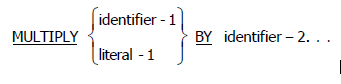## Thursday, 4 September 2014

### COBOL Multiply Statement.COBOL Multiply Statement..

The MULTIPLY statement multiplies numeric items and sets the values of data items equal to the results. Multiply arithmetic statement has two different formats. Refer below the figure for more details.

Format 1:Format 1

Here, the value of identifier-1 or literal-1 is multiplied by the value of identifier-2; the product is then placed in identifier-2. For each successive occurrence of identifier-2, the multiplication takes place in the left-to-right order in which identifier-2 is specified.
Format 2:Format 2
Here, the value of identifier-1 or literal-1 is multiplied by the value of identifier-2 or literal-2. The product is then stored in the data item(s) referenced by identifier-3.
Refer below example for more details.COBOL Multiply ExampleCOBOL Multiply Statement | Share and subscribe to our blog.
-->